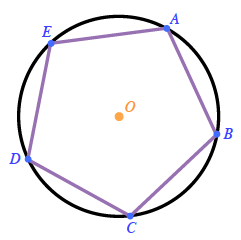### Home > CCG > Chapter Ch10 > Lesson 10.1.2 > Problem10-22

10-22.

$ABCDE$ is a regular pentagon inscribed in $⊙O$, meaning that each of its five vertices just touches the circle.  Homework Help ✎

1. Draw a diagram of $ABCDE$ and $⊙O$ on your paper.2. Find $m∠EDC$. How did you find your answer?

$\text{sum of interior angles}=180(n-2)\\ \qquad \qquad \qquad \qquad \quad \ =180(5-2)$
$\text{sum of interior angles}= 540^{\large\circ}$

$\angle EDC=\frac{1}{5}\text{(sum of interior angles)}$

$\angle EDC=\frac{1}{5}(540)=108^{\large\circ}$

3. Find $m∠BOC$. What relationship did you use?

$\angle BOC=\frac{360^{\large\circ}}{5}=72^{\large\circ}$

4. Find $m\overarc{ E B C }$. Is there more than one way to do this?

How is the inscribed angle related to the intercepted arc?